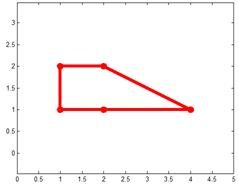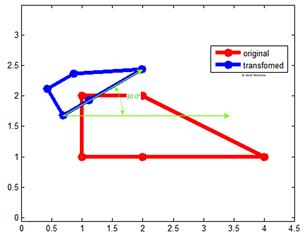Linear Transformation of a Complex Number

Suppose we have a set of complex pointsand we have two complex numbersandthen we can obtain another set of complex pointsusing linear transformationThis complex linear transformation is rigid body transformation which contains a rotation about the origin and a scaling and a translation.

Properties of Complex Linear Transformation

• Complex numbercontrols the scaling by scale ofand the rotation through angle. For positive rotation angle we have,• Complex numbertranslate the results of multiplication ofinto.

Example:

Suppose we have these set of complex points that produce a shape.The plot of Real and Imaginary of the complex points is shown below as a complex plane.We want to design a complex linear transformation that will rotatecounterclockwise and scale it at 0.5 and translate it (0.5, 1)

We want to find the value ofsuch thatand. For the translation, we simply use.

We know thatand.

Thus, we haveand. However, the range of function tan in computer is usually from -pi to +pi, thus, we need to adjust by shifting pi/2 to get the correct result.

From trigonometry, we know that. Thus, we haveandWe input the second equation to the first equation to give-->-->Thus,